# Classification Quiz II, Non Verbal Reasoning

## 20 Questions MCQ Test Non Verbal Reasoning | Classification Quiz II, Non Verbal Reasoning

Description
Attempt Classification Quiz II, Non Verbal Reasoning | 20 questions in 40 minutes | Mock test for LR preparation | Free important questions MCQ to study Non Verbal Reasoning for LR Exam | Download free PDF with solutions
QUESTION: 1

### Directions to Solve In each problem, out of the five figures marked (1), (2), (3), (4) and (5), four are similar in a certain manner. However, one figure is not like the other four. Choose the figure which is different from the rest. Question - Choose the figure which is different from the rest.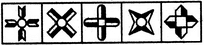(1)     (2)     (3)     (4)     (5)

Solution: All other figures have at least one line of symmetry.
QUESTION: 2

### Directions to Solve In each problem, out of the five figures marked (1), (2), (3), (4) and (5), four are similar in a certain manner. However, one figure is not like the other four. Choose the figure which is different from the rest. Question - Choose the figure which is different from the rest.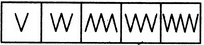(1)     (2)     (3)     (4)     (5)

Solution: Fig. (3) is formed by a combination of A-shaped elements while all other figures are formed by a combination of V-shaped elements.
QUESTION: 3

### Directions to Solve In each problem, out of the five figures marked (1), (2), (3), (4) and (5), four are similar in a certain manner. However, one figure is not like the other four. Choose the figure which is different from the rest. Question - Choose the figure which is different from the rest.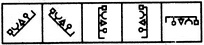(1)     (2)     (3)     (4)     (5)

Solution: All other figures can be rotated into each other.
QUESTION: 4

In each problem, out of the five figures marked (1), (2), (3), (4) and (5), four are similar in a certain manner. However, one figure is not like the other four. Choose the figure which is different from the rest.

Question -

Choose the figure which is different from the rest.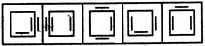(1)     (2)     (3)     (4)     (5)
Solution: In all other figures, the square has two line segments inside and one line segment outside.
QUESTION: 5

In each problem, out of the five figures marked (1), (2), (3), (4) and (5), four are similar in a certain manner. However, one figure is not like the other four. Choose the figure which is different from the rest.

Question -

Choose the figure which is different from the rest.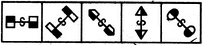(1)     (2)     (3)     (4)     (5)
Solution: Only in fig. (3), the two shaded portions lies on the same side of the main line.
QUESTION: 6

In each problem, out of the five figures marked (1), (2), (3), (4) and (5), four are similar in a certain manner. However, one figure is not like the other four. Choose the figure which is different from the rest.

Question -

Choose the figure which is different from the rest.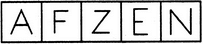(1)     (2)     (3)     (4)     (5)
Solution: Each one of the figures, except fig. (4), is formed by three line segments.
QUESTION: 7

In each problem, out of the five figures marked (1), (2), (3), (4) and (5), four are similar in a certain manner. However, one figure is not like the other four. Choose the figure which is different from the rest.

Question -

Choose the figure which is different from the rest.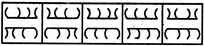(1)     (2)     (3)     (4)     (5)
Solution: In each one of the other four figures, four arcs are curved towards the left and four other acres are curved towards the right.
QUESTION: 8

In each problem, out of the five figures marked (1), (2), (3), (4) and (5), four are similar in a certain manner. However, one figure is not like the other four. Choose the figure which is different from the rest.

Question -

Choose the figure which is different from the rest.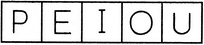(1)     (2)     (3)     (4)     (5)
Solution: All other are vowels.
QUESTION: 9

In each problem, out of the five figures marked (1), (2), (3), (4) and (5), four are similar in a certain manner. However, one figure is not like the other four. Choose the figure which is different from the rest.

Question -

Choose the figure which is different from the rest.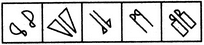(1)     (2)     (3)     (4)     (5)
Solution: All other figures contain a geometrical figure along with its mirror image.
QUESTION: 10

In each problem, out of the five figures marked (1), (2), (3), (4) and (5), four are similar in a certain manner. However, one figure is not like the other four. Choose the figure which is different from the rest.

Question -

Choose the figure which is different from the rest.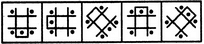(1)     (2)     (3)     (4)     (5)
Solution: All other figures can be rotated into each other.
QUESTION: 11

In each problem, out of the five figures marked (1), (2), (3), (4) and (5), four are similar in a certain manner. However, one figure is not like the other four. Choose the figure which is different from the rest.

Question -

Choose the figure which is different from the rest.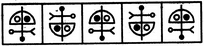(1)     (2)     (3)     (4)     (5)
Solution: All other figures can be rotated into each other.
QUESTION: 12

In each problem, out of the five figures marked (1), (2), (3), (4) and (5), four are similar in a certain manner. However, one figure is not like the other four. Choose the figure which is different from the rest.

Question -

Choose the figure which is different from the rest.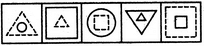(1)     (2)     (3)     (4)     (5)
Solution: In each one of the other figures, one of the two elements is made of dotted lines.
QUESTION: 13

In each problem, out of the five figures marked (1), (2), (3), (4) and (5), four are similar in a certain manner. However, one figure is not like the other four. Choose the figure which is different from the rest.

Question -

Choose the figure which is different from the rest.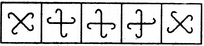(1)     (2)     (3)     (4)     (5)
Solution: All other figures can be rotated into each other.
QUESTION: 14

In each problem, out of the five figures marked (1), (2), (3), (4) and (5), four are similar in a certain manner. However, one figure is not like the other four. Choose the figure which is different from the rest.

Question -

Choose the figure which is different from the rest.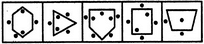(1)     (2)     (3)     (4)     (5)
Solution: In all other figures, the number of dots outside the main figure is one more than the number of dots inside the main figure.
QUESTION: 15

In each problem, out of the five figures marked (1), (2), (3), (4) and (5), four are similar in a certain manner. However, one figure is not like the other four. Choose the figure which is different from the rest.

Question -

Choose the figure which is different from the rest.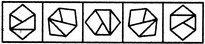(1)     (2)     (3)     (4)     (5)
Solution: All other figures can be rotated into each other.
QUESTION: 16

In each problem, out of the five figures marked (1), (2), (3), (4) and (5), four are similar in a certain manner. However, one figure is not like the other four. Choose the figure which is different from the rest.

Question -

Choose the figure which is different from the rest.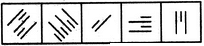(1)     (2)     (3)     (4)     (5)
Solution: In all other figures, all the line segments are drawn perpendicular to one base only.
QUESTION: 17

In each problem, out of the five figures marked (1), (2), (3), (4) and (5), four are similar in a certain manner. However, one figure is not like the other four. Choose the figure which is different from the rest.

Question -

Choose the figure which is different from the rest.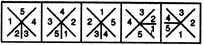(1)     (2)     (3)     (4)     (5)
Solution: Only in fig. (2), while moving in an ACW direction, the numbers do not form a sequence.
QUESTION: 18

In each problem, out of the five figures marked (1), (2), (3), (4) and (5), four are similar in a certain manner. However, one figure is not like the other four. Choose the figure which is different from the rest.

Question -

Choose the figure which is different from the rest.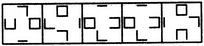(1)     (2)     (3)     (4)     (5)
Solution: Each one of the figures except fig. (2), contains - one complete square, one cup-shaped element having three sides, one 'U-shaped element having two sides and one straight line.
QUESTION: 19

In each problem, out of the five figures marked (1), (2), (3), (4) and (5), four are similar in a certain manner. However, one figure is not like the other four. Choose the figure which is different from the rest.

Question -

Choose the figure which is different from the rest.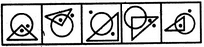(1)     (2)     (3)     (4)     (5)
Solution: In all other figures, one of the dots lies outside the triangle as well as the circle.
QUESTION: 20

In each problem, out of the five figures marked (1), (2), (3), (4) and (5), four are similar in a certain manner. However, one figure is not like the other four. Choose the figure which is different from the rest.

Question -

Choose the figure which is different from the rest.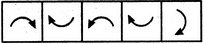(1)     (2)     (3)     (4)     (5)
Solution: In fig. (3), the arrow indicates an ACW motion while in each of the other figures, the arrow indicates a CW motion.Use Code STAYHOME200 and get INR 200 additional OFF Use Coupon Code